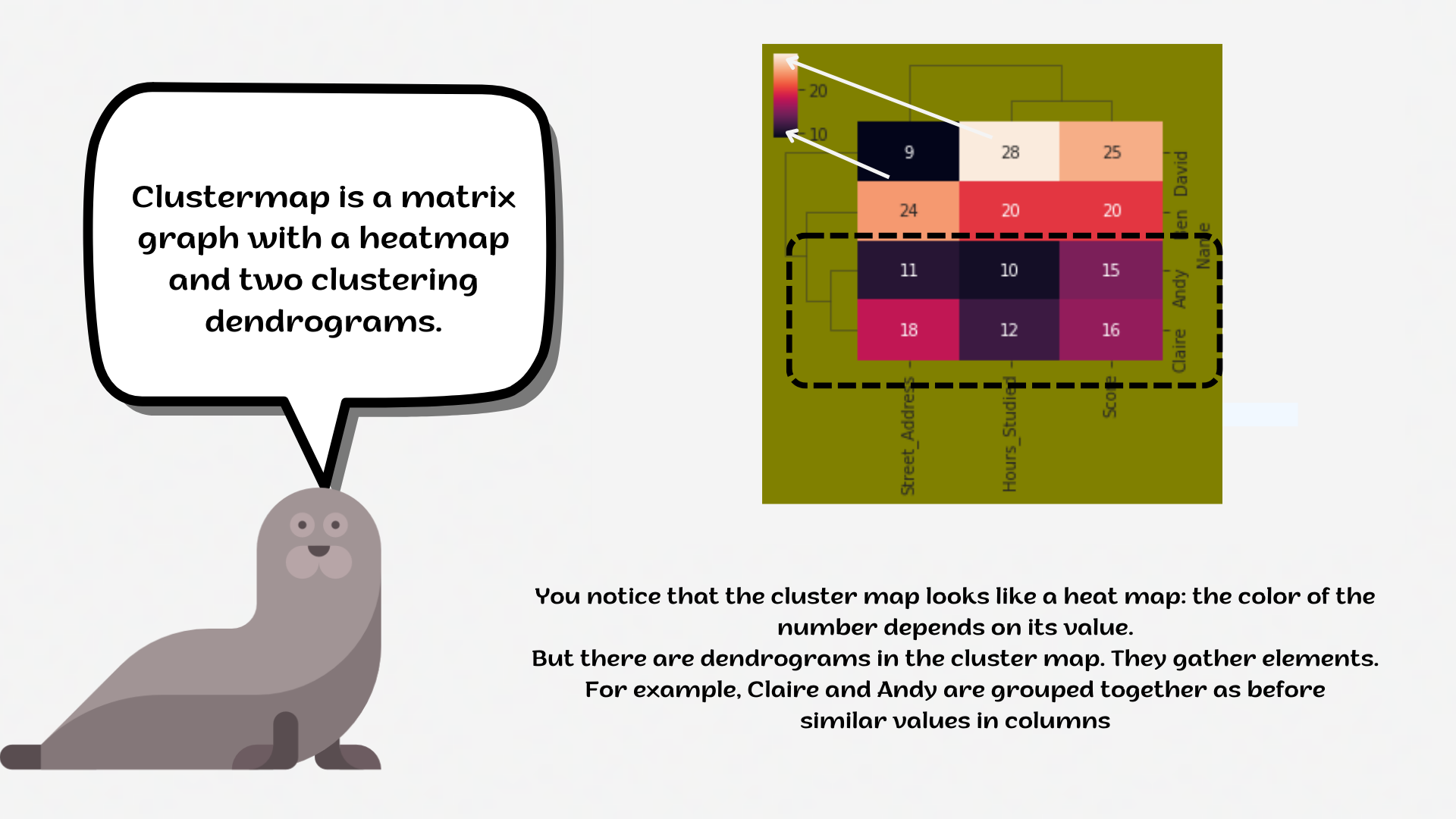Course Content

# Deep Dive into the seaborn Visualization

1. Light Start

2. Distributions of Data

3. Categorical Plot Types

4. Matrix Plots

5. Multi-Plot Grids

6. Regression Models

Deep Dive into the seaborn Visualization

##ClustermapA `clustermap` is a matrix plot with a heatmap and two clustering dendrograms (learn more: Machine Learning).1. Create the `clustermap` using the `seaborn` library:
• Add the data for the `clustermap`. Input only the name of the DataFrame;
• Set the `'vlag'` `cmap`;
• Set the `standard_scale` parameter equals `1`;
• Set the `'single'` `method` parameter;
• Set the `'correlation'` `metric` parameter;
• Add the `annot`;
• Set the `vmin` parameter equals `0` and `vmax` parameter equals `10`;
• Display the plot.

Everything was clear?

Section 4. Chapter 2